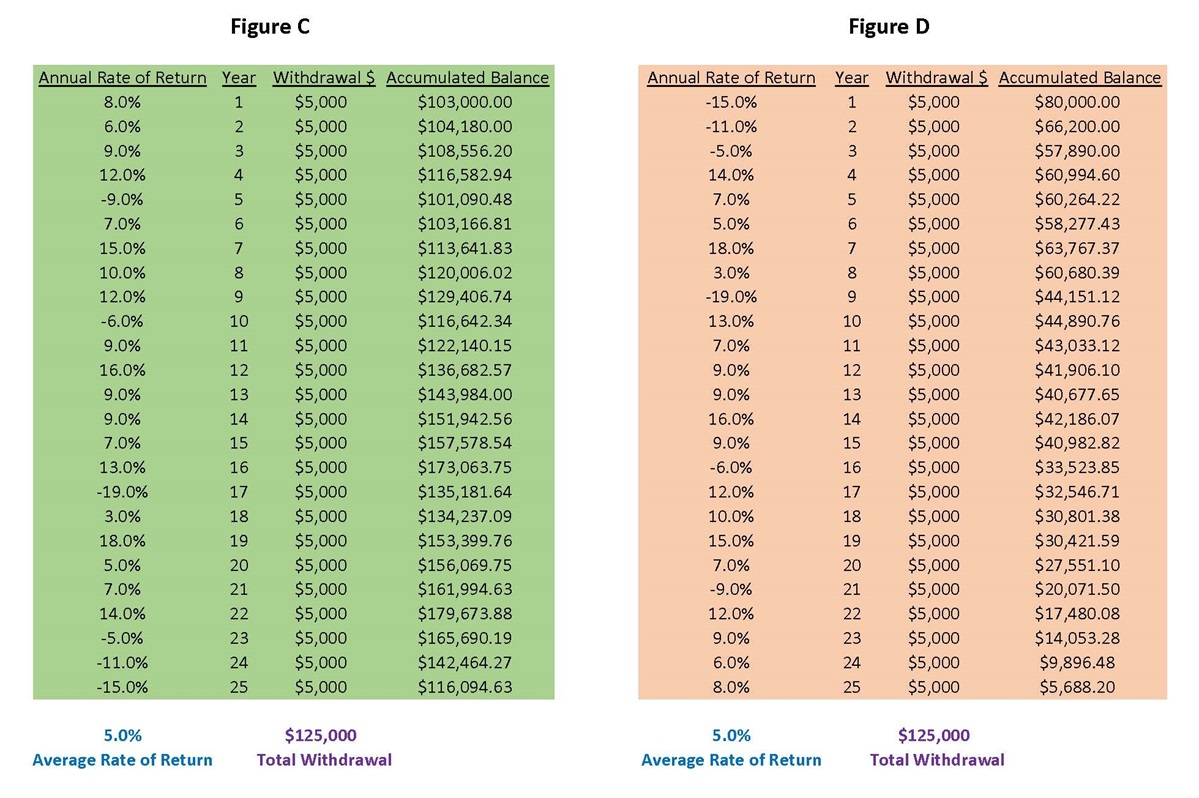## Systematic Withdrawal (or Distribution) Experiences

For this Distribution example let’s assume that same \$100,000 invested with the same hypothetical Average Rate of Return, both reversed sequences of Annual Rates of Return, and a 5% withdrawal rate (\$5000) from the initial investment at the END of each year, starting at the end of Year 1.

Figure C – This reflects a mathematical total withdrawal value of \$125,000 over 25 years with a remaining hypothetical account balance of \$116,094.63.

Figure D – This also reflects a mathematical total withdrawal value of \$125,000 over 25 years but with a remaining account balance of only \$5688.20.Wow, what a difference! What happened?

Even though the hypothetical Average Rate of Return for the full 25 years for both Figures C and D was still 5%, there were positive returns in the early years of Figure C vs negative returns in the early years of Figure D. And that’s what caused the disparity!

But what happens if you factor in inflation? Please click on the systematic "Withdrawals with Inflation" button below to continue.

This is a hypothetical example and is not representative of any specific investment. Your results may vary